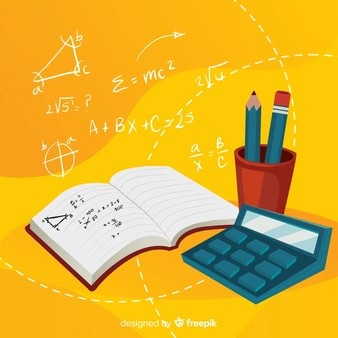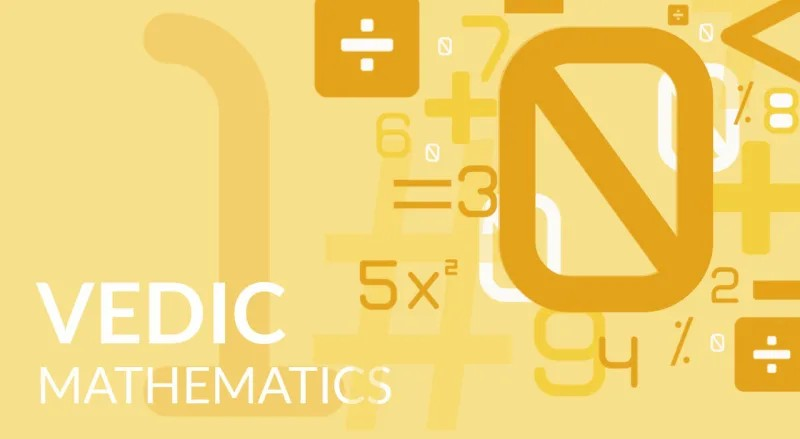# Get the Magical Key to Fast Calculations with Vedic Maths

• 29/10/2020
•

Get the Magical Key to Fast Calculations with Vedic Maths

Vedic mathematics is considered as one of the oldest techniques of learning maths in a simpler way. This method has been used not only by the experts but the kids also since a long time.  It is a huge collection of various ancient techniques including meditation that make the calculations much easier. At the present times, there are numerous ways through which one can improve mathematical skills but Vedic mathematics is one that holds the supreme position.

So, when such a subject is having power all over the earth, let’s see the features of Vedic math that has made it so popular:

Coherence:

The basic of vedic maths is that it has consistency and therefore it is called common core math. Instead of having different techniques, there is uniformity in methods and techniques.

Easy and Fun:

Mostly people say that maths is tough, but the same is not true. Vedic maths formulas and tricks make the subject fun and easy to learn. For example, if you are a beginner to the subject maths, then with vedic maths it is easy to go. Even vedic maths for kids is also taught in many schools and so they can easily learn it with fun and enjoyment.

Flexibility:The modern technique of maths teaching is boring and rigid. But with vedic mathematics there is variation as there are so many special methods which can be applied to solve math problems in the easiest way.

For example if a child is required to solve hard math problems, there will be a great option in the form of vedic mathematics for beginners that will guide him to solve a question with the best math formula and later he can solve any question with a trick of math.

Methods found in Algebra

This is one of the important features of the Vedic maths is that if you have become proficient in an arithmetic method, then that can be applied to algebra formulas of that kind. Some mathematicians have also approved it by applying various basics of Vedic mathematics.

Since this stream of mathematics is bestowed with some of the great features of common core math, it has many benefits. Due to the basic math, its simplicity and the ease of techniques, Vedic maths is gaining popularity worldwide.

Its importance of Vedic maths is not limited to students and kids but to anyone who desires to know and learn maths more easily. Let’s see some of them:

Accurate solving skills:

Vedic calculations which are part of ayurvedic maths increase the accuracy of your math-solving skills. It also has a universal accepted unified system that provides a set of independent cross-checking methods, through which accuracy is 100% sure. The features like flexibility helps in the development of clarity of mind and intuition. This will develop more attentiveness capabilities that will decrease the chance of errors in calculations. You will be a great math problem solver once you are a master of Vedic maths.

Faster in solving problems:

Vedic Maths is a great technique to improve the speed of calculations. With this stream of maths, one can solve the mathematical problems in just a few seconds. It is 10-15 times faster than what is needed in the conventional maths methods. If the techniques of Vedic Maths are understood, there is no need to learn another one as it covers almost all the branches of mathematics like mathematics, geometry and trigonometry formulas. The traditional ways of calculations are burdensome and lengthy, Vedic Mathematics is math stack exchange that helps in solving the problems in a single line solutions.

Improves mental health and intelligence:

Vedic maths is the only branch that sharpens the mind, develops mental agility and intellect. This is the reason it is chosen for the kids at the early stages for learning. It helps in increasing in concentration and visualization of the concepts. At this stage it is just finite math. That is also called mental math as it improves their mental ability to think and solve a particular problem. Taking an example of online vedic classes, where these kids are provided with kindergarten math worksheets to solve through Vedic maths that enhances their mental health and intelligence.

Knowledge and Understanding:Vedic math techniques improve knowledge and understanding of mathematics. It reduces dependence on math calculators. Now-a-days Vedic math methods and its math classes have been a boon for students who are preparing for various examinations that may fit their schooling or any type of competitive exams. Trigonometry problems of any class can be solved with the application of Vedic maths techniques. It not only gives knowledge of our own country but the other country's ancient maths techniques can be learned like Singapore math. It brings out hidden would-be in you and improves overall academic performance.

Creativity & Fun:

Not just calculating abilities, it boosts creativity. It promotes an interest in math and helps to overcome math phobia. Vedic math is fun as it is easy to remember & apply. The tricks are amazing and learning is full of fun. Through snappy maths it is more engaging and fun for the kids.

The learning process of Vedic maths is still on as there are so many maths online classes for it. There are different programs designed for each one like vedic maths for beginners and vedic mathematics class 10 etc. So, get the one best suited to you at MillionCenters Platform and learn the magic of Vedic maths.

#### Who We Are

MillionCenters.com is an online platform to connect learning centers and learners. We make it easy and simple for learners and tutors to connect with each other.

#### Introducing MillionCenters - one app that connects students with teachers and learning centers near them